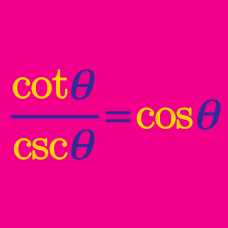Geometry

# Proving Trigonometric Identities: Level 4 Challenges

$\large \tan^2 1^\circ + \tan^2 3^\circ + \tan^2 5^\circ + \cdots+ \tan^2 87^\circ + \tan^2 89^\circ = \ ?$

$\large \sec \left ( \dfrac {\pi}{10} \right ) \sec \left ( \dfrac {3\pi}{10} \right ) \sec \left ( \dfrac {7\pi}{10} \right ) \sec \left ( \dfrac {9\pi}{10} \right ) = \; ?$

$\large \dfrac1{2^x} = \cos (a) \cos(2a) \cos(3a) \cdots \cos(999a)$

The equation above holds true for $a = \dfrac{2\pi}{1999}$. Find $x$.

$\large \tan\frac{\pi}{7}\tan\frac{2\pi}{7}\tan\frac{3\pi}{7}= \sqrt{A}$ Find $A$.

$\large f(x) = \cos(x) \cdot \cos(2x) \cdot \cos(3x)\cdots \cos(999x)$

If $f \left(\dfrac{2\pi }{1999}\right) = \dfrac{1}{2^{k}}$, find $k$.

×